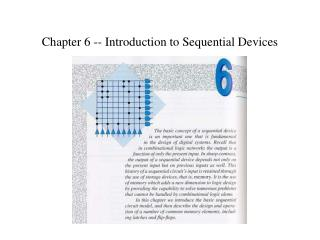Download PresentationChapter 6 -- Introduction to Sequential Devices

Chapter 6 -- Introduction to Sequential Devices - PowerPoint PPT Presentation

Chapter 6 -- Introduction to Sequential Devices. The Sequential Circuit Model. Figure 6.1. State Tables and State Diagrams. Figure 6.2. Sequential Circuit Example. Figure 6.3. Latch and Flip-flop Timing. Figure 6.4. TTL Memory Elements. Set Latch. Figure 6.5. Reset Latch. Figure 6.6.I am the owner, or an agent authorized to act on behalf of the owner, of the copyrighted work described.
Download PresentationChapter 6 -- Introduction to Sequential Devices

Download Policy: Content on the Website is provided to you AS IS for your information and personal use and may not be sold / licensed / shared on other websites without getting consent from its author.While downloading, if for some reason you are not able to download a presentation, the publisher may have deleted the file from their server.

- - - - - - - - - - - - - - - - - - - - - - - - - - E N D - - - - - - - - - - - - - - - - - - - - - - - - - -
Presentation Transcript
1. Chapter 6 -- Introduction to Sequential Devices

2. The Sequential Circuit Model Figure 6.1

3. State Tables and State Diagrams Figure 6.2

4. Sequential Circuit Example Figure 6.3

5. Latch and Flip-flop Timing Figure 6.4

6. TTL Memory Elements

7. Set Latch Figure 6.5

8. Reset Latch Figure 6.6

9. Set-Reset Latch (SR latch) Figure 6.7

10. NAND SR Latch Figure 6.8

11. Set-Reset Latch Timing Diagram Figure 6.9

12. SR Latch Propagation Delays

13. SR Latch Characteristics Figure 6.11 Q* = S + RQ

14. SN74279 Latch with Two Set Inputs Figure 6.12

15. Gated SR Latch Figure 6.13

16. Gated SR Latch Characteristics Figure 6.14 Q* = SC + RQ + C Q

17. Delay Latch (D latch) Figure 6.15

18. D Latch Characteristics Figure 6.16 Q* = DC + CQ

19. D Latch Timing Diagram Figure 6.17

20. D Latch Timing Constraints Figure 6.18

21. The SN74LS75 D Latch Figure 6.19

22. Propagation Delays and Time Constraints for the SN74LS75

23. Hazard-Free D Latch, the SN74116 Figure 6.20 Q* = DC + CQ + DC

24. Master-Slave SR Flip-flop Figure 6.20

25. SR Master-Slave Flip-Flop Characteristics Figure 6.22 Q* = S + RQ

26. Master-Slave D Flip-Flop Figure 6.23

27. Master-Slave D Flip-Flop Characteristics Figure 6.24 Q* = D

28. Pulse-Triggered JK Flip-Flop Characteristics Figure 6.25 Q* = KQ + JQ

29. Pulse-Triggered JK Flip Realization Figure 6.26

30. The SN7476 Dual Pulse-Triggered JK Flip-Flop Figure 6.27

31. SN7474 Excitation Table Figure 6.29

32. SN7474 Flip-Flop Timing Specifications Figure 6.30

33. SN74175 Positive-Edge-Triggered D Flip-Flop Figure 6.31 (a)

34. SN74273 Positive-Edge-Triggered D Flip-Flop Figure 6.31 (b)

35. SN74LS73A Edge-Triggered JK Flip-Flop Logic Diagram Figure 6.32 (a)

36. SN74LS73A Logic Symbols Figure 6.32 (b) and (c)

37. SN74276 and SN74111 Edge-Triggered JK Flip-Flops Figure 6.32 (d) and (e)

38. Negative-Edge-Triggered T Flip-Flop Figure 6.33

39. Edge-Triggered T Flip-Flop Characteristics Figure 6.34 Q* = Q

40. Clocked T Flip-Flop Figure 6.35

41. Excitation Table for Clocked T Flip-Flops Figure 6.36 Q* = TQ + TQ

42. The Clocked T Flip-Flop Timing Diagram Figure 6.37

43. Summary of Latch and Flip-Flop Characteristics

44. SE555 Precision Timing Module Figure 6.38

45. Astable Operation of The SE555 Figure 6.39

46. Monostable (One shot) Device Realization Figure 6.40

47. PROM-based Sequential Circuits Figure 6.41

48. PROM-based Sequential Circuit Example Figure 6.41

49. Prime Number Sequencer Figure 6.43